# GPS Receiver Testing

## Overview

From the airplane pilot operating a Boeing 747, to the everyday consumer using a GPS navigation system in his car, to the hobbyist searching for buried treasure in the forest, GPS technology is quickly becoming integrated in a wide variety of applications. As innovation drives GPS receivers to even better performance, techniques used to characterize performance are becoming increasingly sophisticated as well. Today, with the power of software, you can create GPS waveforms that accurately emulate the real-world signal. In addition, advances in instrument bus technology enable record and playback of live GPS signals with PXI instrumentation.

## Introduction

As GPS technology becomes more commonplace on the commercial market, many designers are working to improve characteristics such as lower power consumption, the tracking of weak satellites, faster acquisition times, and more accurate position fixes. In this application note, learn how to make a variety of GPS receiver measurements including sensitivity, noise figure, position accuracy, time to first fix (TTFF), and position deviation. The goal of this document is to provide engineers with a thorough understanding of GPS measurement techniques. For engineers who are new to GPS receiver measurements, this paper offers a comprehensive overview of common measurements. Engineers who are already experienced at performing GPS measurements can use this document as a resource to introduce new instrumentation technology. This application note is structured according to the following sections:

1.      Basics of GPS Technology
2.      GPS Measurement Systems
3.      Overview of Common Measurements
1. Sensitivity
2. Time to First Fix (TTFF)
3. Position Accuracy and Repeatability
4. Tracking Accuracy and Repeatability

Each section provides several practical tips and techniques. More importantly, you can compare your results to typical results NI engineers have observed from GPS receivers. By correlating your results with both NI and theoretical measurements, you can be sure that your measurement data is valid.

Back to top

## GPS Navigation System

The global positioning system (GPS) is a space-based radio navigation system managed by the U.S. Air Force. While GPS was originally developed as a military positioning system, it has significant benefits for civilian use as well. In fact, it is likely you already use GPS receivers in your car, boat, or even cell phone. The GPS navigation system consists of 24 satellites that transmit multiple message signals in the L1 and L2 frequency bands. In the L1 band, at 1.57542 GHz, each satellite generates a 1.023 Mchips BPSK (binary phase shift keying) spread spectrum signal. The spreading sequence uses a pseudorandom (PN) sequence called the C/A (coarse acquisition) code. Although the spreading sequence is 1.023 Mchips, the actual message data rate is 50 Hz . At the system’s original deployment, GPS receivers were able to achieve a typical accuracy of greater than 30 to 20 m. This level of accuracy was due to an intentional random timing error added by the U.S. military for security reasons. However, on May 2, 2000, the error source (called "selective availability") was removed. Today, receivers are able to achieve better than 5 m of maximum error, with typical errors as low as 1 to 2 m.

In both the L1 and L2 (1.2276 GHz) bands, GPS satellites also generate an additional signal known as the "P code." This signal is a 10.23 Mbits/s BPSK modulated signal that also uses a PN sequence as spreading code. The "P" codes transmitted are used by the military for even greater position precision. In the L1 band, they are transmitted 90 degrees out of phase with the C/A codes to ensure that both can be detected at the same carrier . P codes in the L1 band have a signal power of -163 dBW and a power of -166 dBW in the L2 band. By contrast, the broadcast power for C/A codes in the L1 band is a minimum of -160 dBW on the earth’s surface.

### GPS Navigation Message

For C/A codes, the navigation message consists of 25 frames of data, and each frame contains 1500 bits . In addition, each frame is divided into five 300-bit subframes. With a receiver acquiring C/A codes, it takes exactly six seconds to acquire one subframe and 30 seconds to acquire one frame. Note that the 30 seconds needed to acquire an entire frame actually has profound implications on some of the measurements discussed later in this paper. In fact, the time to first fix (TTFF) measurement is usually greater than 30 seconds because this is the minimum amount of time needed for the receiver to acquire an entire frame.

To achieve a position fix, most receivers must have updated almanac and ephemeris information. This information is contained in the message data transmitted by the satellites, and each subframe contains a unique set of information. Generally, subframes have the following data :

Subframe 1: Clock correction, accuracy, and health information of satellite

Subframes 2-3: The precise orbital parameters used to compute the exact location of each satellite

Subframes 4-5: Coarse satellite orbital data, clock correction, and health information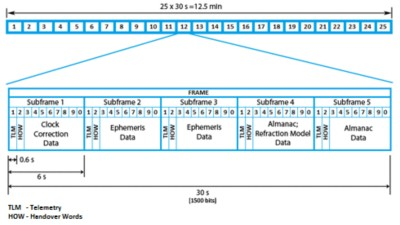Figure 1. Structure of one "frame" of GPS data

Almanac and ephemeris information is critical for the receiver to obtain a position fix. At a high level, GPS receivers return position through a simple triangulation algorithm once the distance to each satellite (pseudorange) is known. In fact, the combination of pseudorange and satellite location information enables the receiver to accurately identify its own position.

Using either the C/A or P codes, receivers are able to achieve a 3D position fix by tracking up to four satellites. While the process of tracking a satellite is quite complex, the basic idea is that the receiver can estimate its position by determining the distance to each tracked satellite. Because signals propagate at the speed of light (c), or 299,792,458 m/s, a receiver can calculate the distance from the satellite, called the "pseudorange," by the following equation: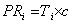Equation 1. Pseudorange as a Function of Time Interval 

The actual process of achieving position fix information occurs by the receiver decoding the message data sent from each satellite. With each satellite broadcasting its unique position, the receiver is able to use the pseudorange difference between each satellite to determine its exact location . Using triangulation, a receiver requires three satellites to achieve a 2D position fix and four satellites to achieve a 3D position fix.

Back to top

## Setting Up a GPS Measurement System

The primary instrument required to test a GPS receiver is an RF vector signal generator that is capable of simulating GPS signals. This application note describes how to use the NI PXIe-5672 RF vector signal generator for this purpose. You can use this instrument with the NI GPS Simulation Toolkit for LabVIEW to generate from one to 12 simultaneous GPS satellites.

The design of a complete GPS measurement system also involves several different accessories to guarantee the best performance. For example, you can use external fixed attenuators to improve power accuracy and noise floor performance. In addition, you may need a DC blocker for some receivers, depending on whether the receiver supplies a DC bias to its direct input port. The complete GPS signal generation system is shown in Figure 1.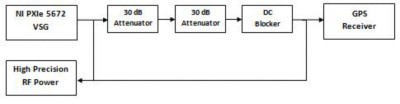Figure 2. Block Diagram of GPS Generation System

You can observe in Figure 2 that up to 60 dB of external RF attenuation (padding) is often used when testing GPS receivers. Fixed attenuators provide the measurement system with at least two benefits. First, they ensure that the noise floor of the test stimulus is well below the thermal noise floor (-174 dBm/Hz). Second, you can use them to improve the power accuracy because you can calibrate signal level with a high-precision RF power meter. While only 20 dB of attenuation is required to meet the noise floor goal, you can achieve best power accuracy and noise floor performance when using 60 to 70 dB of attenuation. Table 1 lists the effect of attenuation on noise floor performance. RF power calibration is discussed in a later section.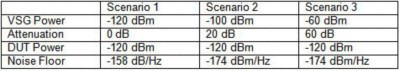Table 1. Comparison of Instrument Power Required for Various Attenuation

As shown in Table 1, you can use attenuation to attenuate noise, but not below the thermal noise floor of -174 dBm/Hz.

### RF Vector Signal Generator

National Instruments recommends the NI PXIe-5672 vector signal generator for GPS test applications. This instrument streams GPS waveforms that are sampled at 1.5 MS/s (I/Q) from disk at a total data rate of 6 MB/s. While you can easily sustain this data rate on a PXI controller hard drive, you should use an external drive for additional storage capacity. Figure 3 shows a typical PXI system with the NI PXIe-5672.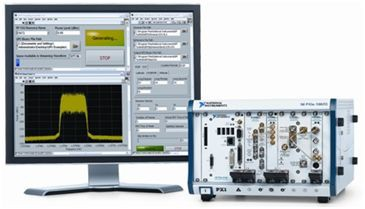Figure 3. PXI System with an NI PXIe-5672 Vector Signal Generator and an NI PXI-5661 Vector Signal Analyzer

With the GPS Simulation Toolkit, you can create waveforms that are up to 12.5 minutes (25 frames) in length, which is the duration of an entire navigation message. Sampled at 6 MB/s, the maximum file size is approximately 7.5 GB. Because of the waveform size, you can store all waveforms on one of several different hard disk options including the following:

• External RAID volumes such as the NI HDD-8263 and HDD-8264
• External USB 2.0 hard disk (tested with Western Digital Passport Hard Drive)

Each of these hard drive configurations is capable of supporting more than 20 MB/s of continuous data streaming. Thus, any of these options enable both simulation and record and playback of GPS signals. A later section in this paper describes how you can use a combination of simulated and recorded GPS waveforms for comprehensive characterization of GPS receiver performance.

### Creating Simulated GPS Signals

Because a GPS receiver uses satellite message data to obtain almanac and ephemeris information, this information is required for simulation of GPS signals as well. Supplied as a text file, almanac and ephemeris data provides information about satellite location, altitude, health, and orbit patterns. In addition, you can use the waveform creation process to select custom parameters such as time of week (TOW), location (longitude-latitude-altitude), and simulated receiver velocity. Based on this information, the toolkit automatically selects up to 12 satellites, calculates all Doppler shift and pseudorange information, and produces the resulting baseband waveform. To help you get started, sample almanac and ephemeris files are included in the toolkit installer. In addition, you can download them directly from the following sites:

With custom almanac and ephemeris files, you can create GPS signals from specific dates and times going back several years. When selecting these files, it is important to choose files that correspond to the same date. In general, almanac and ephemeris information is updated daily, and files from the same day should be used when choosing a specific date and time. Note that ephemeris files are often downloaded in a compressed *.Z format. Thus, you must extract the file with an unzip utility before using it with the GPS Simulation Toolkit.

Using the GPS Simulation Toolkit in “automatic mode,”  where Doppler and pseudorange information is programmatically calculated, covers most GPS simulation use cases, but you can also use the toolkit in manual mode to specify each satellite’s information independently. Table 2 shows the available input parameters for both modes of operation.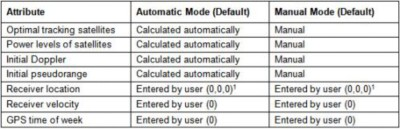1LLA (longitude, latitude, altitude)

Table 2. Default Values for Automatic and Manual GPS Simulation Toolkit Mode

Note the GPS time of week is automatically coerced by the GPS Simulation Toolkit to a range of possible values specified by the ephemeris file. Thus, if a chosen value is out of range of the given ephemeris file, the GPS Simulation Toolkit automatically selects the next-closest possible value and reports a warning to the user. You can use an example program, “niGPS Write Waveform To File,” to create GPS baseband waveforms (automatic mode). Figure 4 shows the front panel.Figure 4. You can create GPS test waveforms with a simple example program.

The specific measurements you choose determine the type of GPS test file that you create. For example, when measuring receiver sensitivity, you should use a single-satellite simulation. On the other hand, measurements that require a position fix (such as TTFF and position accuracy) require you to use a GPS signal that simulates multiple satellites. Because of this, the GPS Simulation Toolkit includes example programs for both single-satellite and multiple-satellite simulations.

### Recording GPS Signals Off the Air

One increasingly common method of creating GPS waveforms is by recording them off the air. In this scenario, signals are recorded with a vector signal analyzer (such as the NI PXI-5661) and the recorded data is generated with a vector signal generator (such as the NI PXIe-5672). Because recording GPS signals enables you to capture real-world signal impairments, you can use signal playback to observe how the receiver will perform in its deployment environment.

You can record GPS signals off the air in a fairly straightforward manner. In an RF recording system, appropriate antennas and amplifiers are combined with a PXI vector signal analyzer and hard disk to capture up to several hours of continuous data. For example, a 2 TB RAID (redundant array of inexpensive disks) is capable of recording up to 25 hours of GPS waveform. In the following sections, explore how to configure an appropriate RF front end for an RF record and playback system.

Each type of wireless communications signal has different requirements for bandwidth, center frequency, and required gain. In the case of GPS, the essential requirement is to record 2.046 MHz of RF bandwidth at a center frequency of 1.57542 GHz. Based on the bandwidth requirements, the sample rate must be at least 2.5 MS/s (1.25 x 2 MHz). Note that the 1.25 multiplier is based on the filter roll-off of the PXI-5661 DDC (digital downconverter) at the decimation stage.

In the tests described below, a sample rate of 5 MS/s (20 MB/s) was used to ensure the entire bandwidth was captured. Because you can achieve data rates of 20 MB/s or more with standard PXI controller hard drives, it is not necessary to use an external RAID volume to stream GPS signals to disk. However, National Instruments recommends using an external hard disk for two reasons. First, you can increase overall storage capacity and record multiple waveforms. Second, the use of external hard disks does not introduce undue stress on the hard drive of the PXI controller. In the tests described below, a USB 2.0 external hard disk was used. This drive, a 320 GB Western Digital Passport, operates at a disk speed of 5400 rpm. During this testing, typical read and write speeds were on the order of 25 to 28 MB/s. Thus, you can use it for both simulated (6 MB/s) and recorded (20 MB/s) GPS waveform data streaming.

The trickiest aspect of recording GPS signals is selecting and configuring the appropriate antenna and low-noise amplifier (LNA). Observe that with a typical passive patch antenna, the typical peak power in the L1 GPS band ranges from -120 to -110 dBm (the tests showed power at -116 dBm). Because the power level of GPS signals is so small, significant amplification is required to ensure that the vector signal analyzer can capture the full dynamic range of the satellite signals. While there are several ways to apply the appropriate level of gain to the signal, you can achieve the best results when using an active GPS antenna with the NI PXI-5690 preamplifier. With two cascaded LNAs, each providing 30 dB of gain, the total gain applied is 60 dB (30 + 30). Thus, the resulting peak power observed by the vector signal analyzer is increased from -116 to -56 dBm. An example system using this configuration is shown in Figure 5.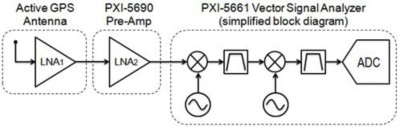Figure 5. GPS receivers implement cascaded LNAs.

Note that one essential requirement of the recording system is the active GPS antenna. An active GPS antenna combines a patch antenna and an LNA into a single package. These antennas typically require a DC bias voltage of 2.5 to 5 V, and you can easily purchase them off-the-shelf for about \$20 USD. For simplicity, use one with an SMA connector. The following section shows how the noise figure of the first LNA in an RF front end is crucial to ensuring that the recording instrumentation adds as little noise as possible to the off-the-air signal. Also note that the vector signal analyzer shown in Figure 5 is a simplified diagram. The actual PXI-5661 is a three-stage super-heterodyne vector signal analyzer that is slightly more complex than the illustration in Figure 5.

When you apply 60 dB to the off-the-air signal, you should observe the peak power in the L1 at about -60 to -50 dBm. If you configure the vector signal analyzer in swept spectrum mode to analyze the entire spectrum, note that the power in bands outside the L1 band (FM and cellular) rise to power levels that are actually higher than the GPS signal. However, the peak power of out-of-band signals does not typically exceed -20 dBm, and is filtered by one of the vector signal analyzer's several bandpass filters. One of the easiest ways to verify that the RF front end of the recording device is sufficient is by opening the RFSA demo panel example program. Using this program, you can visualize the RF spectrum at the L1 GPS band. A typical view of the spectrum is shown in Figure 6. Note that this spectrum screenshot was taken outdoors at the GPS center frequency. An active GPS antenna and PXI-5690 preamplifier were used to apply a combined total of 60 dB of gain.

Center Frequency: 1.57542 GHz

Span: 4 MHz

RBW: 10 Hz

Averaging: RMS, 20 Averages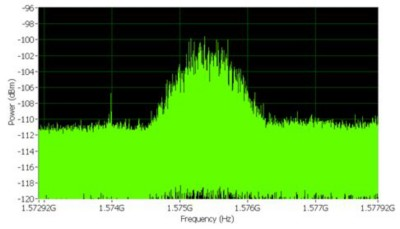Figure 6. GPS is visible only in the spectrum with a narrow RBW.

Using an RF record and playback NI LabVIEW example program discussed earlier, configure the reference level to -50 dBm, the center frequency to 1.57542 GHz, and the I/Q sample rate to 5 MS/s. A front panel of a configured example is shown in the Figure 7.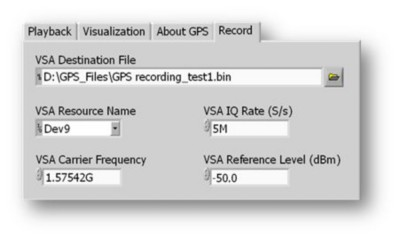Figure 7. Front Panel of RF Record and Playback Example

The maximum recording duration of a GPS signal depends on the sample rate and the maximum storage capacity. Using a 2 TB RAID volume (the largest addressable disk size in Windows XP), you can record signals at 5 MS/s for up to 25 hours.

### Configuring the RF Front End

With cascaded LNAs providing 60 dB of gain, you significantly increase the power at the front end of the vector signal analyzer. From your measurement, 60 dB of gain was enough to increase the peak power from -116 dBm to -56 dBm. Note that with 60 dB of gain applied (and a 1.5 dB noise figure), the noise power of the signal is –112 dBm/Hz (-174 + Gain + F). The maximum obtainable signal-to-noise ratio (SNR) of the signal, 56.5 dB (-56 dBm +112.5 dBm), is less than the dynamic range of the instrument, so you can be sure that with 80 dB of dynamic range, your vector signal analyzer can record the maximum possible SNR without introducing noise in the off-the-air signal.

When recording any signal off the air, it is a good practice to set the reference level at least 5 dB above the typical peak power to account for any signal strength anomalies. While this reduces the effective dynamic range of the vector signal analyzer in some cases, GPS signals are unaffected by this technique. Because the maximum theoretical SNR of a GPS signal at the antenna input is 58 dB (-116 + 174), you gain no advantage by recording more than 58 dB of dynamic range at the vector signal analyzer. Thus, you can essentially “throw away” 10 dB or more of your instrument’s dynamic range without affecting the quality of the recorded signal (at this bandwidth, the PXI-5661 has a dynamic range of better than 75 dB).

With the reference level appropriately set, you need to properly configure the RF front end of the recording device. As previously mentioned, you can achieve the best RF recording results using an active GPS antenna. While the active antenna uses a built-in LNA to provide up to 30 dB of gain with a low noise figure, you must also supply it with a DC bias. Several biasing methods are described below.

##### Method 1: Active Antenna Powered by GPS Receiver

The first method to power an active antenna is with a DC bias "T." Using this component, a DC signal (3.3 V in this case) is applied to the DC port of the bias T, which applies the appropriate DC offset to the active antenna. Note that the precise DC voltage you should apply depends on the DC power requirements of the active antenna. A diagram illustrating the connections is shown in Figure 8.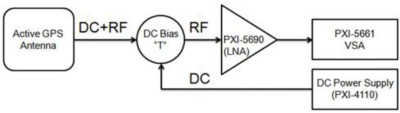Figure 8. You can use a DC bias "T" to power an active GPS antenna.

Observe in Figure 8 that you can use an NI PXI-4110 programmable DC power supply to supply the DC bias signal. While you can use many off-the shelf power supplies (including many less expensive ones) for this application, the PXI-4110 was used in this case as a matter of convenience. Also, you can use a generic off-the-shelf bias T that is operational up to 1.58 GHz, but the one used in this experiment was purchased from www.minicircuits.com.

##### Method 2: Active GPS Antenna Powered by Receiver

A second method that you can use to power the active GPS antenna is with the receiver itself. Most off-the-shelf GPS receivers use a single port to power an active GPS antenna, and this port is already biased with an appropriate DC signal. By combining an active GPS receiver with a splitter and DC blocker, you can power an active LNA and simply record the signal observed by the GPS receiver. A diagram of the appropriate connections is shown in Figure 9.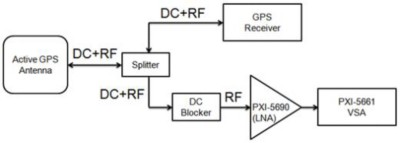Figure 9. With a DC blocker, you can record and analyze the GPS signal.

Figure 9 shows how you can use DC bias from the GPS receiver to power the LNA. Note that method 2 is particularly useful for drive tests because you can observe the receiver’s characteristics such as velocity and dilution of precision while recording.

#### Cascaded Noise Figure Calculations

To calculate the total noise that is added to the recorded GPS signal, you can simply determine the noise figure for the entire RF front end. As a matter of principle, the noise figure of the entire system is always dominated by the first amplifier in the system. Think of noise figure as the ratio of SNRin to SNRout (see Noise Figure for measurement techniques) through any RF component or system. When recording GPS signals, it is necessary to determine the noise figure of the entire RF front end.

When performing a cascaded noise figure calculation, you first convert each noise figure and gain to its linear equivalent, which is called the "noise factor." When calculating the noise figure for a system with cascaded RF components, you can first determine the system noise factor and then convert to noise figure. Thus, you must calculate system noise figure using the following equation: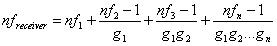Equation 2. Noise Figure Calculation for Cascaded RF Amplifiers 

Note that both noise factor (nf) and gain (g) are shown in lowercase because they are linear and not logarithmic relationships. Thus, you also introduce the conversion from linear to logarithmic gain and noise figure (and vice versa) in the equations below: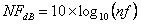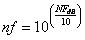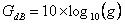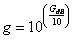Equations 3 through 6. Conversions between Linear and Logarithmic Gain and Noise Figure 

An active GPS antenna using a built-in LNA typically provides 30 dB of gain while introducing a noise figure usually on the order of 1.5 dB. During the second stage of the recording instrumentation, the PXI-5690 provides 30 dB of additional gain as well. Though its noise figure is higher (5 dB), the second amplifier introduces little noise into the system. As an academic exercise, you can use equation 2 to calculate the noise factor for the entire RF front end of the recording instrumentation. Gain and noise figure values are expressed in Table 3.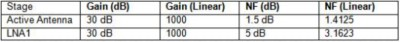Table 3. Noise Figure and Factors of the First Two Components of the RF Front End

According to the calculations above, you can determine the overall noise factor for the receiver: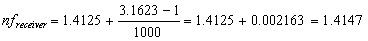Equation 7. Cascaded Noise Figure for an RF Recording System

To convert noise factor into a noise figure (in dB), you apply Equation 3 to yield the following results: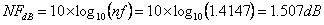Equation 8.  The noise figure of the first LNA dominates the noise figure of the receiver.

As Equation 8 illustrates, the noise figure of the first LNA (1.5 dB) dominates the noise figure of the entire measurement system. Thus, with the vector signal analyzer configured so that the noise floor of the instrument is less than that of the input stimulus, your recording introduces only 1.507 dB of noise to the off-the-air signal.

### Talking to the GPS Receiver

While many receivers may use proprietary software that enables the user to visualize information such as longitude and latitude, a more standardized approach is required for automated measurements. Fortunately, you can configure a wide variety of receivers to talk to a PXI controller through a protocol known as NMEA-183. In this case, the receiver continuously sends commands through either a serial or USB cable. In LabVIEW software, you can parse all commands to return satellite and position fix information. The NMEA-183 protocol supports six basic commands, and each provides unique information. These commands are described in Table 4.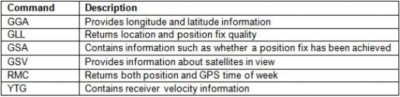Table 4. Overview of Basic NMEA-183 Commands

For practical testing purposes, the GGA, GSA, and GSV commands are the most useful. More specifically, you can use information from the GSA command to determine whether the receiver has achieved a position fix and is used in TTFF measurements. When performing sensitivity measurements, use the GSV command to return C/N (carrier-to-noise) ratios for each satellite that you are tracking.

This application note does not describe the NMEA-183 protocol in great depth, but you can find all command information at various Web sites such as www.gpsinformation.org/dale/nmea.htm#RMC. In LabVIEW, you can parse these commands using the NI-VISA driver.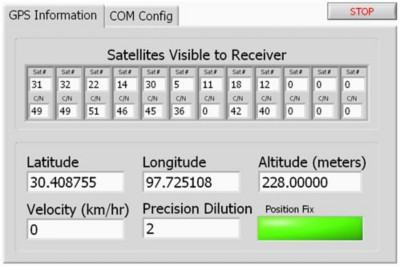Figure 10. LabVIEW Example Using NMEA-183 Protocol

Back to top

## GPS Measurement Techniques

While you can use a wide variety of measurements to characterize the performance of a GPS receiver, several common measurements apply to all GPS receivers. This section examines the theory and practice of performing measurements such as sensitivity, TTFF, position accuracy/repeatability, and position tracking uncertainty. Note that you can use many different methods to validate position accuracy and perform functional test of receiver tracking ability. This section describes several basic methods, but they are by no means the complete set.

Back to top

## Introduction to Sensitivity Measurements

Sensitivity is one of the most important measurements of a GPS receiver’s capability. In fact, for many commercial-grade GPS receivers, it is often the only RF measurement performed in production test of the final product. At a high level, the sensitivity measurement defines the lowest satellite power level at which a receiver is still able to track and achieve a position fix on satellites overhead. As you might expect, GPS receivers are required to apply significant gain through several cascaded LNAs to amplify the signal to the appropriate power level. Unfortunately, while an LNA  increases signal power, it degrades SNR. Thus, as the RF power levels of a GPS signal increase, SNR decreases and eventually the receiver is no longer able to track the satellite.

Many GPS receivers actually specify two sensitivity values: acquisition sensitivity and signal tracking sensitivity . As the names suggest, acquisition sensitivity represents the lowest power level at which a receiver is able to achieve a position fix. By contrast, signal tracking sensitivity is the lowest power level at which a receiver is able to track an individual satellite.

Fundamentally, you can define sensitivity as the lowest power level at which any wireless receiver produces a desired minimum bit error rate (BER). Because BER is well-correlated with C/N ratio, sensitivity is often measured by validating  the C/N ratio reported by the receiver at a known input power level.

Note that the C/N ratios for each satellite are directly reported by the GPS receiver chipset. You can calculate this value several ways – some receivers actually approximate it by calculating a BER of the message date. Modern GPS receivers typically report a peak C/N in the range of 54 to 56 dB-Hz when stimulated with a high-power test stimulus. The C/N limit is fitting because even with a clear view of the sky, a GPS receiver is likely to report C/N values ranging from 30 to 50 dB-Hz. For typical GPS receivers, the minimum C/N ratio required to achieve a position fix (acquisition sensitivity) ranges from 28 to 32 dB-Hz. Thus, for a particular receiver, you can define sensitivity as the minimum power level required for the receiver to produce the minimum position fix C/N ratio.

In theory, you can measure sensitivity with either a single-satellite or multisatellite test stimulus. In practice, this measurement is performed most commonly with single-satellite test because RF power can be more easily and more reliably determined. By definition, sensitivity is the lowest power level at which a receiver returns a desired minimum C/N ratio. In the next section, learn how a receiver’s sensitivity is highly dependent on the noise figure of the RF front end. Mathematically, you can relate sensitivity to the noise figure of the receiver according to the following equation: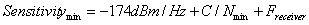Equation 9. Sensitivity as a Function of C/N and Noise Figure

Equation 9 shows how you can express sensitivity as a function of both C/N ratio and noise figure. As an example, if your minimum C/N required for position tracking is 32 dB-Hz, a receiver with a noise figure of 2 dB has a sensitivity of -140 dBm (-174 + 32 + 2). However, when testing the baseband transceiver alone, the first LNA is often bypassed. A typical receiver is illustrated in Figure 11.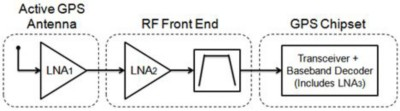Figure 11. GPS receivers often cascade several LNAs. 

As you can see, a typical GPS receiver actually cascades several LNAs to provide sufficient gain to the GPS signal. The first LNA dominates the noise figure for the entire system. In Table 5, assume that LNA1 has a gain of 30 dB and an NF of 1.5 dB. In addition, the entire RF front end has a gain of 40 dB and an NF of 5 dB. Note that because the noise power after LNA2 exceeds thermal noise of -174 dBm/Hz, the bandpass filter attenuates both signal and noise. As a result, it has little effect on SNR. Finally, assume that the GPS chipset produces a gain of 40 dB with a noise figure of 5 dB. You can calculate the noise figure of the entire system.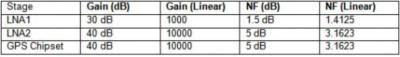Table 5. Gain and NF in Both Linear and Logarithmic Form

According to the calculations above, you can determine the overall noise factor for the receiver: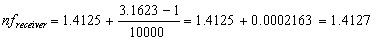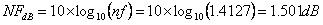Equations 10 and 11. The noise figure of the first LNA dominates the noise figure of the receiver.

From equations 10 and 11, you can determine that your GPS receiver with an active antenna connected has a noise figure of approximately 1.5 dB. Note that you ignored the third term in the cascaded noise figure equation. Because this value is so small, you can essentially eliminate the term.

In some cases, a GPS receiver uses an active antenna with a built-in LNA. Thus, the test point bypasses the first LNA of the receiver. In this case, the noise figure is dominated by the second LNA, which often has a greater noise figure than that of the first. If you remove LNA1, you can calculate the noise figure from LNA2 looking into the receiver with the following equation: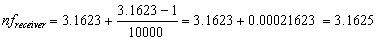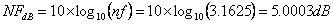Equations 12 and 13. Noise Figure of a Receiver with First LNA Removed

As equations 12 and 13 illustrate, removing the LNA with the best noise figure significantly affects the noise figure for the entire receiver. Note that while this exercise in calculating the noise figure for a “typical” GPS receiver is purely theoretical, it is nonetheless important. Because the receiver’s reported C/N ratio is highly dependent on the noise figure of the system, knowing the system’s noise figure can help you set appropriate C/N test limits.

### Single-Satellite Sensitivity Measurement

Now that you understand the basic theory of the sensitivity measurement, this section helps you explore the process of performing an actual measurement. In a typical test system, a simulated L1 single-satellite carrier is fed into the RF port of the DUT through a direct connection. To report the C/N ratio, establish that your receiver is configured to communicate via the NMEA-183 protocol. In LabVIEW, you simply read the maximum reported satellite C/N from parsing the three GSV commands.

According to the GPS specification documents, the power of a single L1 satellite should be no less than -130 dBm at the earth's surface . However, consumer demands to use GPS receivers indoors or in urban environments have pushed the typical test limits much lower. In fact, many GPS receivers report position tracking sensitivity down to -142 dBm and signal tracking down to -160 dBm. Most GPS receivers can maintain the lock of a signal 6 dB below the typical operating point very quickly, so, in this example, use an average RF power level of -136 dBm for your test stimulus.

For best power accuracy and noise floor performance, National Instruments recommends the use of external attenuation at the output of the RF vector signal generator. In most scenarios, 40 to 60 dB of external attenuation is sufficient to operate the generator in a more linear region (power ≥ -80 dBm). Because the fix attenuation of each pad contains some uncertainty, you must first calibrate your system to determine the exact power of the test stimulus.

In this calibration phase, you can account for signal peak-to-average ratio, part-to-part variation of attenuators, and insertion loss of any cabling used. To calibrate the system, disconnect cabling from the DUT and reconnect the exact same cable to an RF vector signal analyzer such as the PXI-5661.

### Part A: Single-Satellite Calibration

When performing sensitivity measurements, RF power-level accuracy is one of the most important characteristics of the signal generator. Because receivers report C/N to within 0 digits of precision (in other words, 34 dB-Hz), sensitivity measurements in production test are made within ±0.5 dB of power accuracy. Thus, it is important to ensure that your instrumentation has equal or better performance. Because general-purpose RF instrumentation is specified for operation across a broad range of power levels, frequency ranges, and temperature conditions, you can often achieve measurement repeatability that is much better than the specified instrument performance by implementing a basic system calibration. The following section provides insight into two methods that you can use to guarantee the best RF power accuracy.

#### Method 1: Single Passive RF Attenuator

Although use of external attenuation is required to ensure the best noise density for GPS signal generation, only 20 dB of attenuation is actually required to ensure that the noise density is below -174 dBm/Hz. When using a 20 dB fixed pad, simply program your instrument to an RF power level that is 20 dB above the desired level. To hit your target of -136 dBm, program the instrument to -115 dBm (assuming 1 dB cable insertion loss) and connect the 20 dB attenuator directly to the output of the generator. The resulting RF power is -136 dBm but with added uncertainty. Assuming your 20 dB fixed pad has an uncertainty of ±0.25 dB, and the RF generator has an uncertainty of ±1.0 dB at -116 dBm, the overall uncertainty is ±1.25 dB. Thus, while method 1 is the simplest approach and does not require calibration, the use of multiple components in the system without calibration introduces substantial uncertainty. One of the greatest contributors to instrument uncertainty is VSWR, or voltage standing wave ratio. With a passive attenuator connected directly to the output of the instrument, the standing wave reflected back to the instrument is attenuated as well. By reducing one of the greatest contributors to power uncertainty, you improve overall power accuracy.

You also can use a high-end vector network analyzer (VNA) to measure the exact passive attenuation. Using this measurement device, you can determine the exact attenuation applied by the pad to within ±0.1 dB of uncertainty.

#### Method 2: Multiple Passive Attenuators with Calibration

A second method for calibrating RF power is to use a high-precision RF power meter (measurements better than ±0.2 dB accuracy down to -70 dBm) with a series of fixed attenuators. Because you are operating the RF generator at a fixed frequency and over a relatively small power range, you can effectively calibrate any error introduced by the generator. In addition, because passive attenuators operate with linear behavior at a fixed frequency, you can calibrate their uncertainty. With method 2, the key to ensuring the best performance is to configure a generation system with as little uncertainty as possible. Using a high-precision power meter with better than 80 dB of dynamic range (often a dual-head instrument), you can ensure the best measurement uncertainty.

A high-precision power meter helps you calibrate the system with three measurements: one for the RF power of the vector signal generator and two measurements to calibrate the attenuators. To achieve the best uncertainty, you should configure a system that requires the least number of measurements. For a resulting RF power level of -136 dBm, you can program the RF instrument to a power level of -65 dBm and use 70 dB of fixed attenuation (assuming 1 dB insertion loss). To determine the exact RF power level that you should program, you can calibrate the attenuation achieved through fixed padding. The calibration process is as follows:

1)     Program the vector signal generator to a power level of +15 dBm

To do this, open the NI Measurement & Automation Explorer configuration utility and use the test panels. With the test panel, generate a 1.58 GHz continuous wave (CW) signal at +15 dBm.

2)     Measure RF power with a precision power meter

Using the precision RF power meter, observe that the power is +14.78 dBm (or similar), which is within the instrument’s power accuracy specifications.

3)     Attach 70 dB fixed attenuators (30 dB + 20 dB + 20 dB) and any cabling

4)     Measure RF power with a precision power meter

With the power meter configured to the maximum number of averages (512), measure the RF power level. Your reading is -56.63 dBm.

5)     Calculate total RF loss

By subtracting -56.63 dBm from +14.78 dBm, you can determine that the combination of attenuators and cabling  introduces 71.41 dB of power loss. Note that many attenuators are often specified to have an uncertainty of up to ±1.0 dB. Thus, the measured attenuation can vary by as much as ±3.0 dB. It is important to calibrate a series of attenuators to ensure that the exact attenuation is known with less uncertainty.

Based on the calibration routine for the attenuators and cabling, you can next determine the RF power level required to achieve -136 dBm. With 71.41 dB of attenuation introduced, you need to program the RF vector signal generator to a power level of -58.59 dBm. To verify that the programmed power is as expected, follow the steps listed below.

6)     Attach the precision power meter directly to the RF vector signal generator

All attenuators and cabling are removed for this step.

7)     Program the RF generator to the value necessary for a final power of -136 dBm

The programmed value should be -58.59 dBm, which is -136 dBm + 71.41 dB.

8)     Measure the resulting power with a power meter

The measured RF power can vary in accordance with the power accuracy of your instrument. While -58.59 was measured, actual results vary slightly from one instrument to the next per the uncertainty of the instrument.

9)     Adjust the generator power until the power meter reads -58.59 dBm

Although the RF generator operates within the tolerance of the specification, this value is repeatable and can be calibrated by adjusting the RF power until the appropriate value is measured.

Using the method described above, you can determine the resulting RF power with only three RF power measurements. Thus, assuming your measurement device has an uncertainty of ±0.2 dB, you can be certain that the power uncertainty at -136 dBm is ±0.6 dBm  (3 x 0.2).

### Part B: Sensitivity Measurement

Now that you have calibrated the power of your RF measurement system, you can measure sensitivity by programming your RF generator to the power level at which you expect the receiver to return the minimum C/N. While the exact RF power used to measure sensitivity varies from one receiver to the next, the ratio of the receiver of C/N to RF power is perfectly linear. In your test, you can assume that the required C/N ratio is 28 dB-Hz to achieve a position fix. From Equation 12, you can derive a relationship between the C/N ratio of the receiver and its noise figure.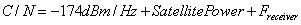Equation 14. C/N as a Function of Noise Figure and Satellite Power

Assuming a constant satellite power, you can observe that the C/N ratio reported by the receiver is merely a function of the noise figure of the receiver. Various achievable C/N ratios are listed in Table 6.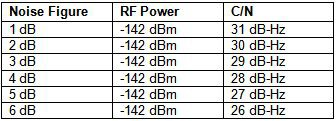Table 6. C/N as a Function of Noise Figure

Generally, the GPS decoding chipset on a receiver determines the minimum C/N ratio required to achieve a position fix. However, it is the noise figure of the entire receiver that determines the C/N ratio that you can achieve at a given power level. Thus, when measuring sensitivity, it is important to know the minimum C/N ratio required to achieve a position fix.

You have several options to measure sensitivity. Table 6 shows that RF power is directly correlated with sensitivity. Thus, you can either measure the receiver’s C/N ratio at the given sensitivity power level or you can derive sensitivity based on RF power at a different power level.

To illustrate this point, consider the relationship between RF signal power and a GPS receiver’s C/N ratio for various power levels. Note that the measurements shown in Table 7 were made by applying a stimulus that bypassed the first LNA, and that the overall receiver’s noise figure is approximately 8 dB.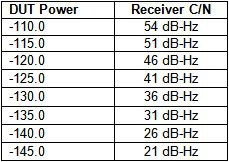Table 7. Receiver C/N as a Function of RF Power

As Table 7 shows, the example measurements suggest a completely linear relationship between RF power and C/N ratio. The one exception occurs when a high input power is used to stimulate the C/N ratio, in which case the receiver reports the maximum possible C/N value. However, these results are expected because the chipset used for the experiment does not report C/N values greater than 54 dB-Hz in any conditions.

Based on the linear relationship between RF power and sensitivity in Table 6, you can conduct production test of a GPS receiver by stimulating the receiver at a variety of power levels. If the receiver reports a C/N value of 28 dB-Hz at -142 dBm, it also reports a C/N value of 34 dB-Hz at -136 dBm. In scenarios where measurement speed is important, you can use a higher C/N value and extrapolate the sensitivity information from the result.

### Determining Noise Figure

Equations 13 and 14 show that you can also determine the noise figure of the receiver or chipset based on the reported C/N ratio. This is reflected in Equation 15.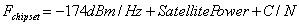Equation 15. Receiver Noise Figure as a Function of Power and C/N Ratio

Table 7 shows that the noise figure of a receiver is directly proportional to the RF power level and C/N ratio. Based on this relationship, you can measure the chipset’s noise figure by correlating the RF power level with the C/N ratio. In this measurement, you increase the generator’s power in 0.1 dB increments. Because the NMEA-183 protocol reports satellite C/N to the nearest decimal digit, estimating noise figure beyond one digit of precision requires you to investigate the C/N rounding of the receiver. Example results are shown in Table 8.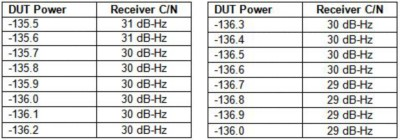Table 8. Correlation of DUT Power and Receiver C/N

Table 8 results show that RF power levels between -136.6 and -135.7 dBm all yield the same C/N ratio of 30 dB-Hz. Based on the rounding principles involved when reporting NMEA-183 data, it is safe to assume that a power level of -136.1 dBm produces a C/N ratio of 30.0 dB-Hz. Using Equation 14, the chipset’s noise figure is therefore -174.0 dBm + -136.1 dBm + 30.0 dB-Hz = 7.9 dB. Note that this calculation is dependent on two uncertainty factors: the power uncertainty of the vector signal generator and the C/N uncertainty reported by the receiver.

Back to top

## Multisatellite GPS Receiver Measurements

While sensitivity measurements require a single-satellite stimulus, many other receiver measurements need a test stimulus that simulates multiple satellites. More specifically, measurements such as TTFF, position accuracy, and dilution of precision all require the receiver to obtain a position fix. Because a receiver needs at least four satellites to obtain a 3D position fix, each of these measurements takes longer than the sensitivity measurements. As a result, many position fix measurements are performed in validation and verification and not in production test.

This section examines two methods to provide the receiver with a multisatellite signal. In the discussion of GPS simulation, learn how to perform TTFF and position accuracy measurements. In the discussion on RF record and playback, examine techniques to validate receiver performance over a broad range of environmental conditions.

### Measuring Time to First Fix (TTFF) and Position Accuracy

TTFF and position accuracy measurements are most important in the design validation stage of a GPS receiver. In many consumer GPS applications, the time it takes for the receiver to return its actual location can significantly affect the receiver’s usability. In addition, the accuracy with which a receiver returns its reported location is important.

For a receiver to obtain a position fix, it must download the almanac and ephemeris information from the satellite through a navigation message. Because it takes 30 seconds for a receiver to download an entire GPS frame, a “cold start” TTFF condition can take anywhere from 30 to 60 seconds. In fact, many receivers specify several TTFF conditions. The most common are the following:

Cold Start: The receiver must download almanac and ephemeris information to achieve a position fix. Because at least one GPS frame must be downloaded from each of the satellites, most modern receivers achieve a position fix from a cold start condition in 30 to 60 seconds.

Warm Start: The receiver has some almanac information that is less than one week old but does not have any ephemeris information. Typically, the receiver knows the time to within 20 seconds and the position to within 100 km . Most modern GPS receivers achieve a position fix from a warm condition in less than 60 seconds but can sometimes achieve a position fix in much less time.

Hot Start: A hot start occurs when a receiver has up-to-date almanac and ephemeris information. In this scenario, the receiver needs to obtain only timing information from each satellite to return its position fix location. Most modern GPS receivers return a position fix from a hot start condition within 0.5 to 20 seconds.

In most cases, TTFF and position accuracy are specified at a specific power level. It is valuable to verify the accuracy of both of these specifications under a variety of circumstances. Because GPS satellites circle the earth every 12 hours, the range of available satellites varies substantially even throughout the course of one day to ensure that your receiver returns the appropriate result under a broad range of conditions.

The next section explains how to perform both TTFF and position accuracy measurements using two sources of data from the following list: (1) live data where the receiver is set up in its deployment environment with an antenna, (2) recorded data where a receiver is tested with an RF signal that was recorded off the air, and (3) simulated data where an RF generator is used to simulate the exact time of week when live data was recorded. By testing a receiver with three different sources of data, you can verify that your measurements from each source are both repeatable and correlated with other data sources.

### Measurement Setup

For best results, choose a recording location where satellites are least obstructed by surrounding buildings. In this case, the top floor of a six-story parking deck provides a sufficient view of the sky and access to as many satellites as possible. You can perform the TTFF measurement using various start modes of the GPS chipset. For example, you can use the SIRFstarIII chipset to reset the receiver for factory, cold, warm, or hot start modes. The measurements shown below are the result of tests performed using this receiver.

To measure horizontal position accuracy, you must determine the error based on the reported latitude and longitude coordinates. Because these figures are reported in degrees, you can convert to meters with the following approximation: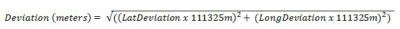Equation 16. Calculating GPS Position Error

The equation above 111,325 m (111.325 km) is equivalent to one degree (of 360) rotation around the earth. This figure is based on the calculation of the earth’s circumference being 360 x 111.325 km = 40.077 km.

#### Off-the-Air GPS

Measuring a receiver’s TTFF in an off-the-air scenario, where the receiver is directly connected to an antenna, is the least precise measurement; however, it is important because it allows you to calibrate the automated measurements made from recorded and simulated GPS signals. You can program the SIRFstarIII chipset into a mode that places the receiver into a cold-start scenario and make all the measurements using the TTFF values reported by the receiver. The GPS receiver used in this case had a specified cold-start TTFF time of 32.6 seconds. Table 9 shows the results.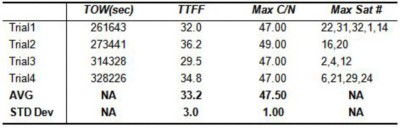Table 9. TTFF and Maximum C/N for Off-the-Air GPS Signals

Based on the initial off-the-air results, you can observe that your GPS receiver is capable of achieving TTFF of 33.2 with a standard deviation of 3.0 seconds. These measurements are within reasonable tolerance of the chipset’s TTFF specification. More importantly, however, you can compare this measurement with results achieved through both simulated and recorded GPS data.

Using the equation for linear deviation above, you can calculate the linear standard deviation of each measurement from the mean position.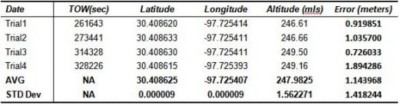Table 10. Reported LLA for Off-the-Air GPS Signals

Note that to correlate off-the-air GPS signals with simulated and playback signals, it is important to correlate the power of the off-the-air signal. When making TTFF and position accuracy measurements, the exact RF power level does not substantially affect the result. Thus, it is sufficient to generally correlate RF power by matching the C/N ratio of off-the-air, simulated, and recorded GPS signals.

#### Recorded GPS Signals

While you can measure TTFF and position deviation with live signals, these measurements are often nonrepeatable because satellites are constantly orbiting the earth. One technique for obtaining repeatable TTFF and position accuracy measurements is with recorded GPS signals. Thus, this section discusses how to correlate live GPS signals with recorded GPS signals.

You can regenerate recorded GPS signals using an RF vector signal generator. Upon playback, the easiest way to calibrate the RF power level is by matching the live C/N ratio with the recorded C/N ratio. When observing off-the-air signals, notice the peak C/N of between 47 and 49 dB-Hz for all live signals.

At a playback power level that results in the same C/N ratio as the live signal, you can be certain that the reported TTFF and position accuracy is well-correlated with that of the live signal. In Table 11, TTFF results are reported for four different trials at time-of-week (TOW) values that are similar with the live off-the-air signal.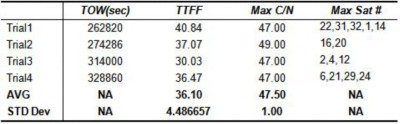Table 11. Reported TTFF for Off-the-Air GPS Signals

In addition to measuring TTFF, you can measure the latitude, longitude, and altitude reported by the GPS receiver. Results are shown in Table 12.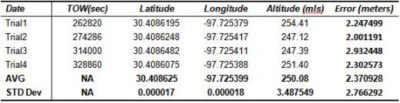Table 12. Reported LLA for Off-the-Air GPS Signals

From the results in tables 11 and 12, you can achieve reasonably repeatable TTFF and LLA (latitude, longitude, altitude) results using recorded GPS signals. However, note that the error and standard deviation for each of these measurements is slightly larger than for the off-the-air measurements. However, while the absolute accuracy is larger, the repeatability is better than with off-the-air measurements.

### Simulated GPS Signals

A final source of GPS test signals for TTFF and position accuracy measurements is simulated multisatellite GPS signals. With the NI GPS Simulation Toolkit for LabVIEW, you can simulate up to 12 satellites at user-defined TOW, week number, and receiver location. The primary benefit of this method of GPS signal simulation is that it results in a GPS signal with the best possible SNR. In fact, unlike live and recorded GPS signals, you can create repeatable signals where the noise power is extremely small. To illustrate this, the frequency domain of a simulated multisatellite signal is shown in Figure 12.

Vector Signal Analyzer Settings

Center: 1.57542 GHz

Span: 4 MHz

RBW: 100 Hz

Averaging: RMS, 20 Average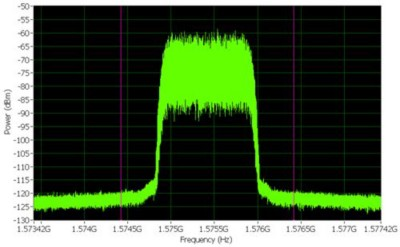Figure 12. Power-in-Band Measurement of a Simulated Multisatellite GPS Signal

When testing a receiver with a simulated multisatellite waveform, you can again estimate the required RF power by correlating the receiver’s reported C/N ratio.

Once you have properly correlated the RF power level, you can measure TTFF. When measuring TTFF, first start the RF vector signal generator. After five seconds, manually place the receiver into “cold” start mode. Once the receiver obtains a position fix, it reports the TTFF information. Results for the simulated GPS signal are shown in Table 13.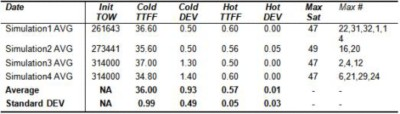Table 13. TTFF Values for Four Unique Simulations

All of the simulations in Table 13 have the same LLA (latitude, longitude, and altitude).

In addition to measuring TTFF, you can calculate LLA accuracy and repeatability by creating simulations at various TOWs. It is crucial to test accuracy at various TOWs because the available satellites change substantially even over the course of several hours (shown in Table 13). The resulting latitude, longitude, and altitude information is shown in Table 14.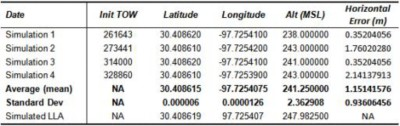Table 14. Horizontal Accuracy for Various TOW Simulations

Table 14 shows that you can calculate horizontal error in meters absolutely based on the simulated position. The horizontal error shown in Table 14 is determined from Equation 17.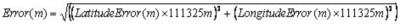Equation 17. Position Error for Simulated GPS Signals

For the receiver used in these experiments, the maximum horizontal position error is 5.2 m and the average horizontal position error is 1.5 m. Thus, the results from Table 14 illustrate that the receiver is well within the specified limits.

As mentioned earlier, the accuracy that a receiver is likely to attain is highly dependent on the available satellites that it has to lock to. Thus, while a receiver’s accuracy is likely to vary substantially over the course of several hours (when satellites change), the repeatability from one run to the next is generally quite small. To verify that this is the case with your GPS receiver, you can perform multiple trials of a particular simulated GPS waveform. This is done primarily to verify that the RF instrumentation does not add uncertainty to the simulated GPS signal. As you can see in Table 15, the example GPS receiver reports highly repeatable measurements when the same binary file is used over and over again.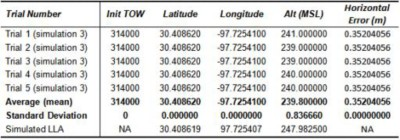Table 15. Error is highly repeatable for each trial of the same waveform.

One of the greatest benefits of using simulated GPS signals is that they help us achieve repeatable position results. This is highly important in the design validation stage of development because it helps you make sure the reported position does not vary from one design iteration to the next.

### Measuring Dynamic Position Accuracy

Another method of GPS receiver testing is measuring the receiver’s tracking ability to maintain a position fix at a wide range of power levels and velocities. Historically, one common approach to this type of testing (often merely a functional test) is with a combination of drive testing and multipath fading emulation. In a drive test, a prototype receiver is simply driven through a route that is known for introducing significant signal impairments. While the drive test is a simple way to apply natural impairments to GPS satellite signals, these measurements are often nonrepeatable. In fact, the combination of factors such as movement in GPS satellites, changes in weather conditions, and even time of year can affect a receiver’s performance.

Thus, one increasingly common method to validate receiver performance in a scenario with significant signal impairments is by recording the GPS signal on a drive test. For more details on how to configure a GPS recording system, see the earlier section. Note that with a drive test scenario, there are several PXI chassis options. The simplest option is to use a DC chassis powered by the car battery. A second option is to use the standard AC chassis with an inverter used to power the chassis off the car’s power supply. Between these two options, the DC chassis consumes less power, but it also is more difficult to power back in the lab. A standard AC chassis, powered off a system consisting of an extra car battery plus a DC-to-AC inverter, generated the results shown below.

Once you have completed your GPS signal recording, you can test the receiver repeatedly with the same set of test data. The experiments below tracked the receiver’s latitude, longitude, and velocity over time. Data was read from the receiver using a serial port, and NMEA-183 commands were read at a rate of once per second. The measurements shown below report receiver characteristics such as position and satellite C/N ratios. You also can perform these measurements while analyzing other information. Horizontal dilution of precision (HDOP) was not measured in the experiment below, but this characteristic provides significant information about a receiver’s position fix accuracy.

For the best results, you should tightly synchronize the command interface of the receiver with the RF generation. The results below show that the RF vector signal generator was synchronized with the GPS receiver by using the data line of the COM port (pin 2) as a start trigger. Using this synchronization method, the vector signal generator and GPS receiver are synchronized to within one clock cycle of the arbitrary waveform generator (100 MS/s). Thus, the maximum skew should be 10 µs. Because you are reporting the latitude and longitude of the receiver, the inaccuracy induced by synchronization error is 10 µs x max velocity (m/s), or 0.15 mm.

Using the configuration described above, you can report the receiver’s latitude and longitude over time. Figure 13 illustrates the results.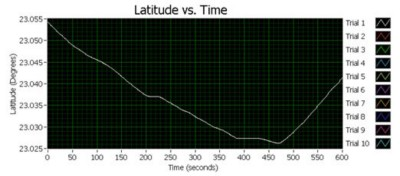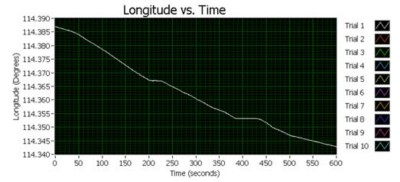Figure 13. Receiver Latitude and Longitude over the Course of a Four-Minute Span

As Figure 13 shows, a recorded drive test signal reports static, position, and velocity information. In addition, you can observe that this information is relatively repeatable from one trial to the next – as evidenced by the difficulty in graphically observing each trace. In fact, you are most interested in the repeatability for the receiver. Because repeatability information offers an estimate of how a GPS receiver’s accuracy changes over time, you also can compute the standard deviation between each sample in the waveforms above. Figure 14 shows the standard deviation of position (relative to mean position) between each synchronized sample over time.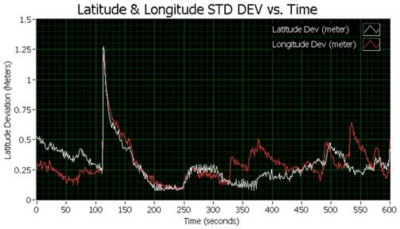Figure 14. Standard Deviation of Both Latitude and Latitude over Time

When observing the horizontal standard deviation, note that the standard deviation appears to rapidly increase at time = 120 seconds. To investigate this phenomenon further, plot the total horizontal standard deviation against the receiver’s velocity (m/s) and a proxy for C/N ratio. Assume that the satellite C/N ratio affects only the receiver in the condition that there is no high-power satellite. Thus, you graph a proxy for C/N by averaging the C/N ratios for the four highest satellites reported by the receiver. Figure 14 illustrates the results.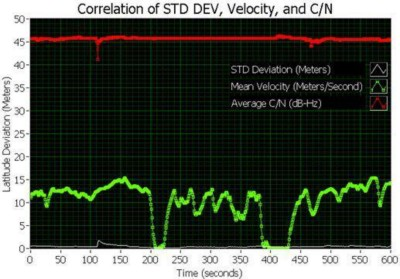Figure 15. Correlation of Position Accuracy and C/N Ratio

Figure 15 shows that the peak horizontal error (in standard deviation) occurring at time = 120 seconds is directly correlated with satellite C/N ratios and not correlated with receiver velocity. At this sample, the standard deviation is nearly 2 m, while it is less than 1 m throughout most other times. Concurrently, you see the top four C/N averages drop from nearly 45 dB-Hz to 41 dB-Hz.

The exercise above illustrates not only the effect of C/N ratio on position accuracy but also the types of analysis that you can conduct using recorded GPS data. For the experiment above, the drive recording of the GPS signal was actually conducted in Huizhou, China (a city north of Shenzhen). However, the actual receiver was tested at a later date in Austin, Texas.

Back to top

## Conclusion

As you have seen from the techniques described above, you can choose from a variety of methods to test GPS receivers. While basic measurements such as sensitivity are almost always used in production test, you also can apply measurement techniques to validate a receiver’s performance. These testing techniques are varied, but you can perform each method of testing within a single PXI system. In fact, you can test GPS receivers with both simulated and recorded baseband waveforms. With a combined approach, you can perform a comprehensive measurement of GPS receiver functionality, from sensitivity to repeatability tracking.

### References

 Pratt, Bostonian, and Allnutt. Satellite Communications.

 Navstar GPS User Equipment Introduction, September 1996.

 Gu, Quzheng, RF System Design of Transceivers for Wireless Communications, Springer, 2005. Fundamentals.

 Ward, Phillip W., Betz, John W., and Hegarty, Christopher J. Chapter 5: Satellite Signal Acquisition, Tracking and Data Demodulation, excerpt from: Understanding GPS: Principles and Applications by Elliot D. Kaplan, Artech House, 2005.

 Global Positioning System: Theory and Applications, Edited by Bradford W. Parkinson and James J. Spilker.

 Braasch, Michael S. and Van Dierendonck, A. J. GPS Receiver Architectures and Measurements, Proceedings of the IEEE, 1999.

 Global Positioning System Standard Positioning Service Signal Specification, 1995.

 Global Positioning System Standard Positioning Service Signal Specification. Annex A, Standard Positing Service Performance Specification, 1995.

 Goldberg, Hans-Joachim. Atmel White Paper: Measuring GPS Sensitivity, 2007.

Back to top